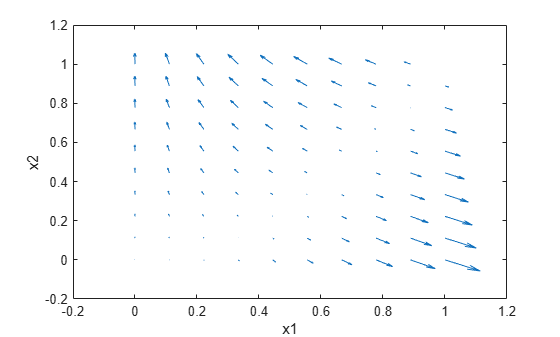Compute gradients for custom training loops using automatic differentiation

## Syntax

``[dydx1,...,dydxk] = dlgradient(y,x1,...,xk)``
``[dydx1,...,dydxk] = dlgradient(y,x1,...,xk,'RetainData',true)``

## Description

Use `dlgradient` to compute derivatives using automatic differentiation for custom training loops.

### Tip

For most deep learning tasks, you can use a pretrained network and adapt it to your own data. For an example showing how to use transfer learning to retrain a convolutional neural network to classify a new set of images, see Train Deep Learning Network to Classify New Images. Alternatively, you can create and train networks from scratch using `layerGraph` objects with the `trainNetwork` and `trainingOptions` functions.

If the `trainingOptions` function does not provide the training options that you need for your task, then you can create a custom training loop using automatic differentiation. To learn more, see Define Custom Training Loops.

example

````[dydx1,...,dydxk] = dlgradient(y,x1,...,xk)` returns the gradients of `y` with respect to the variables `x1` through `xk`.Call `dlgradient` from inside a function passed to `dlfeval`. See Compute Gradient Using Automatic Differentiation and Use Automatic Differentiation In Deep Learning Toolbox.```
````[dydx1,...,dydxk] = dlgradient(y,x1,...,xk,'RetainData',true)` causes the gradient to retain intermediate values for reuse in subsequent `dlgradient` calls. This syntax can save time, but uses more memory. See Tips.```

## Examples

collapse all

Rosenbrock's function is a standard test function for optimization. The `rosenbrock.m` helper function computes the function value and uses automatic differentiation to compute its gradient.

`type rosenbrock.m`
```function [y,dydx] = rosenbrock(x) y = 100*(x(2) - x(1).^2).^2 + (1 - x(1)).^2; dydx = dlgradient(y,x); end ```

To evaluate Rosenbrock's function and its gradient at the point `[–1,2]`, create a `dlarray` of the point and then call `dlfeval` on the function handle `@rosenbrock`.

```x0 = dlarray([-1,2]); [fval,gradval] = dlfeval(@rosenbrock,x0)```
```fval = 1×1 dlarray 104 ```
```gradval = 1×2 dlarray 396 200 ```

Alternatively, define Rosenbrock's function as a function of two inputs, `x1` and x`2`.

`type rosenbrock2.m`
```function [y,dydx1,dydx2] = rosenbrock2(x1,x2) y = 100*(x2 - x1.^2).^2 + (1 - x1).^2; [dydx1,dydx2] = dlgradient(y,x1,x2); end ```

Call `dlfeval` to evaluate `rosenbrock2` on two `dlarray` arguments representing the inputs `–1` and `2`.

```x1 = dlarray(-1); x2 = dlarray(2); [fval,dydx1,dydx2] = dlfeval(@rosenbrock2,x1,x2)```
```fval = 1×1 dlarray 104 ```
```dydx1 = 1×1 dlarray 396 ```
```dydx2 = 1×1 dlarray 200 ```

Plot the gradient of Rosenbrock's function for several points in the unit square. First, initialize the arrays representing the evaluation points and the output of the function.

```[X1 X2] = meshgrid(linspace(0,1,10)); X1 = dlarray(X1(:)); X2 = dlarray(X2(:)); Y = dlarray(zeros(size(X1))); DYDX1 = Y; DYDX2 = Y;```

Evaluate the function in a loop. Plot the result using `quiver`.

```for i = 1:length(X1) [Y(i),DYDX1(i),DYDX2(i)] = dlfeval(@rosenbrock2,X1(i),X2(i)); end quiver(extractdata(X1),extractdata(X2),extractdata(DYDX1),extractdata(DYDX2)) xlabel('x1') ylabel('x2')```## Input Arguments

collapse all

Variable to differentiate, specified as a scalar `dlarray` object. For differentiation, `y` must be a traced function of `dlarray` inputs (see Traced dlarray) and must consist of supported functions for `dlarray` (see List of Functions with dlarray Support).

Example: `100*(x(2) - x(1).^2).^2 + (1 - x(1)).^2`

Example: `relu(X)`

Variable in the function, specified as a `dlarray` object, a cell array, structure, or table containing `dlarray` objects, or any combination of such arguments recursively. For example, an argument can be a cell array containing a cell array that contains a structure containing `dlarray` objects.

If you specify `x1,...,xk` as a table, the table must contain the following variables:

• `Layer` — Layer name, specified as a string scalar.

• `Parameter` — Parameter name, specified as a string scalar.

• `Value` — Value of parameter, specified as a cell array containing a `dlarray`.

Example: `dlarray([1 2;3 4])`

Data Types: `single` | `double` | `logical` | `struct` | `cell`

Indicator for retaining trace data during the function call, specified as `false` or `true`. When this argument is `false`, a `dlarray` discards the derivative trace immediately after computing a derivative. When this argument is `true`, a `dlarray` retains the derivative trace until the end of the `dlfeval` function call that evaluates the `dlgradient`. The `true` setting is useful only when the `dlfeval` call contains more than one `dlgradient` call. The `true` setting causes the software to use more memory, but can save time when multiple `dlgradient` calls use at least part of the same trace.

Example: `dydx = dlgradient(y,x,'RetainData',true)`

Data Types: `logical`

## Output Arguments

collapse all

Gradient, returned as a `dlarray` object, or a cell array, structure, or table containing `dlarray` objects, or any combination of such arguments recursively. The size and data type of `dydx1,...,dydxk` are the same as those of the associated input variable `x1,…,xk`.

collapse all

### Traced `dlarray`

During the computation of a function, a `dlarray` internally records the steps taken in a trace, enabling reverse mode automatic differentiation. The trace occurs within a `dlfeval` call. See Automatic Differentiation Background.

## Tips

• `dlgradient` does not support higher order derivatives. In other words, you cannot pass the output of a `dlgradient` call into another `dlgradient` call.

• A `dlgradient` call must be inside a function. To obtain a numeric value of a gradient, you must evaluate the function using `dlfeval`, and the argument to the function must be a `dlarray`. See Use Automatic Differentiation In Deep Learning Toolbox.

• To enable the correct evaluation of gradients, the `y` argument must use only supported functions for `dlarray`. See List of Functions with dlarray Support.

• If you set the `'RetainData'` name-value pair argument to `true`, the software preserves tracing for the duration of the `dlfeval` function call instead of erasing the trace immediately after the derivative computation. This preservation can cause a subsequent `dlgradient` call within the same `dlfeval` call to be executed faster, but uses more memory. For example, in training an adversarial network, the `'RetainData'` setting is useful because the two networks share data and functions during training. See Train Generative Adversarial Network (GAN).

﻿